Wednesday 25th November 2020
 CBSE Guess > Papers > Question Papers > Class XII > 2005 > Physics > Outside Delhi Set-III PHYSICS 2005 (Set III—Outside Delhi)
Except for the following questions all the remaining questions have been asked in Set I & Set II. Q. 1. Two electric field lines never cross each other. Why? 1 Q. 2. Under what conditions is the force acting on a charge moving through a uniform magnetic field minimum?1 Q. 3. What is the power dissipated in an a.c circuit an which voltage and current are given by 1 Q. 4. In an experiment on photoelectric effect, the following graphs were obtained between the photoelectric current ( I ) and the anode potential (V). Name the characteristic of the incident radiation that was kept constant in this experiment. 1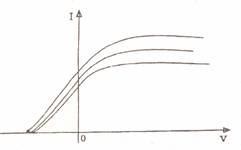Q. 5. How does the effective power radiated by an antenna vary with wave length? 1 Q. 7. Mention the factors on which the resonant frequency of a series LCR circuit depends. Plot a graph showing variation of impedance of a series LCR circuit with the frequency of the applied a.c. source. 2 Q. 10. How do you convert a galvanometer into an ammeter? Why is an ammeter always connected in series? 2 Q. 11. Two fixed point charges -Me and +e units are separated by a distance 'a'. Where should the third point charge be placed for it to be in equilibrium? 2 Or A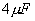capacitor is charged by a 200 V supply. The supply is then disconnected and the charged capacitor is connected to another uncharged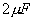capacitor. How much electrostatic energy of the first capacitor is lost in the process of attaining the steady situation? Q. 17. A double convex lens made of glass of refractrive index 1.5 has its both surfaces of equal radii of curvature of 20 cm each. An object of 5 cm height is placed at a distance of 10 cm from the lens. Find the position, nature and size of the image. 3 Q. 22. The half-life of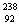U against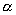-decay is 4.5 X 109 years. Calculate the activity of 1 g sample of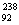U. Physics 2005 Question Papers Class XII Delhi Outside Delhi Compartment Delhi Compartment Outside Delhi Set I Set I Set I Set I Set II Set II Set II Set II Set III Set III Set III CBSE 2005 Question Papers Class XII